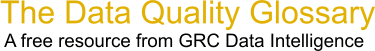Choose Index below for a list of all words and phrases defined in this glossary.

# Hypothesis Testing

index | Index

## Hypothesis Testing - definition(s)

Hypothesis Testing - Hypothesis testing refers to the process of using statistical analysis to determine if the observed differences between two or more samples are due to random chance (as stated in the null hypothesis) or to true differences in the samples (as stated in the alternate hypothesis). A null hypothesis (H0) is a stated assumption that there is no difference in parameters (mean, variance, DPMO) for two or more populations. The alternate hypothesis (Ha) is a statement that the observed difference or relationship between two populations is real and not the result of chance or an error in sampling. Hypothesis testing is the process of using a variety of statistical tools to analyze data and, ultimately, to fail to reject or reject the null hypothesis. From a practical point of view, finding statistical evidence that the null hypothesis is false allows you to reject the null hypothesis and accept the alternate hypothesis.

[Category=Data Quality ]

Source: iSixSigma, 26 January 2011 08:11:13, http://www.isixsigma.com/index.php?option=com_glossaryData Quality Glossary.  A free resource from GRC Data Intelligence. For comments, questions or feedback: dqglossary@grcdi.nl